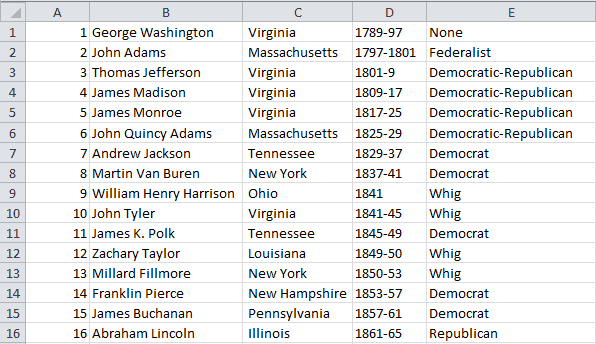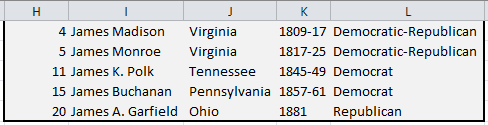Introduction | Example | Tutorial | Applications

## Introduction - VBA InStr

The VBA InStr function is one of the most useful string manipulation functions around. You can use it to test if a string, cell or range contains the substring you want to find. If it does, “InStr” will return the position in the text string where your substring begins. This is great if you are looping through a file or spreadsheet and want to take an action when you find a matching string.

Keep reading to find out how you can use InStr in your VBA macros to search for a substring! I’ll provide all the examples you could possibly want to help get you started.

## Example - VBA InStr

Let’s say you have a list of names in an Excel spreadsheet or text file. Beside each name, you have information about that person. It could be anything - email addresses, phone numbers, employee IDs, countries. This is a common structure for spreadsheets or databases.

Your boss asks you to summarize information about every person that meets a certain criteria. The VBA InStr function is great at handling tasks like these.

For this example, I’m going to start with a list of US Presidents.VBA InStr Tutorial Data

Yes, I understand this is a silly example because you can just use regular Excel filtering to solve that problem, but where’s the fun in that? Familiarizing yourself with VBA InStr allows you to do so much more than simple filtering.

Let’s find all the instances of Presidents with “James” in their name and summarize the results:

### Find Substrings using InStr

``````Sub InStrDemo()
Dim lastrow As Long
Dim i As Integer, icount As Integer

lastrow = ActiveSheet.Range("A30000").End(xlUp).Row
For i = 1 To lastrow
If InStr(1, LCase(Range("B" & i)), "james") <> 0 Then
icount = icount + 1
Range("H" & icount & ":L" & icount) = Range("A" & i & ":E" & i).Value
End If
Next i
End Sub``````

Make powerful macros with our free VBA Developer Kit

This is actually pretty neat. If you have trouble understanding or remembering it, our free VBA Developer Kit can help. It’s loaded with VBA shortcuts to help you make your own macros like this one - we’ll send a copy, along with our Big Book of Excel VBA Macros, to your email address below.

I'll take a free VBA Developer Kit

This macro loops through the entire column of data and finds strings that contain the substring “james” by using the InStr function. Once it finds a match, it copies the data to a separate portion of the Excel spreadsheet.VBA InStr Tutorial Results

If you’re ready to learn more, the following sections show you how InStr works and how you can use it to do more helpful tasks - more examples below!

## Tutorial - VBA InStr

### InStr Arguments

The `InStr` VBA function accepts 4 arguments, 2 of which are optional:

``InStr([Start], MainString, SubString, [Compare As VbCompareMethod])``

Here’s a description of the arguments:

Start [Optional] This is the positive integer position where you want your string to begin searching for SubString inside MainString. If omitted, it starts from the beginning (position 1). A value less than or equal to 0 yields an error.

MainString The string you want to search within. You want to search for SubString inside of MainString.

SubString The substring you want to search for. You want to search for SubString inside of MainString

Compare        [Optional] This tells VBA which algorithm to use when comparing. There are three possible choices, described below.

Here are the three possible VbCompareMethods for the Compare argument:

1. vbBinaryCompare (Default)
2. vbDatabaseCompare
3. vbTextCompare

The default, vbBinaryCompare, is adequate for the vast majority of applications, so I’m not going to waste time explaining each algorithm. Leave a comment in the comments section if you want me to dive into more detail.

### InStr Fundamentals

Now that we got the arguments out of the way, let’s talk fundamentals.

InStr returns an Integer - actually a Variant of type Long - showing where the substring occurs in the main string.

If the substring does not exist, it returns a value of 0. That’s why InStr is so useful! If you only want to take an action when a string contains a substring and you don’t care where the substring is you just check to see if the InStr result does not equal 0.

``If InStr(MainString, SubString) <> 0 Then``

The InStr vbBinaryCompare is case-sensitive. If you want to search for a substring inside a main string and you’re not worried about case sensitivity, it’s a good practice to wrap your MainString and SubString arguments in the LCase function. You could use the vbTextCompare algorithm, but it’s a little buggy. It’s especially buggy when handling rare characters.

``If InStr(LCase(MainString), LCase(SubString)) <> 0 Then``

Subscribe below, then take a look at these InStr Examples to better illustrate these fundamentals. They start off simple, but get more advanced as you go.

### More InStr Examples

#### Return the Substring Position using InStr

##### Example 1
``i = InStr("This is a test", "test")``

The variable i is 11.

##### Example 2
``i = InStr("This is a test", "t")``

The variable i is 11.

##### Example 3
``i = InStr("This is a test", "T")``

The variable i is 1.

##### Example 4
``i = InStr(1, LCase("This is a test"), "t")``

The variable i is 1 because you converted your main string to lowercase.

##### Example 5
``i = InStr("This is a test", "s")``

The variable i is 4.

##### Example 6
``i = InStr(5, "This is a test", "s")``

The variable i is 7 because you started your search at position 5 - after the first “s.”

##### Example 7
``i = InStr(100, "This is a test", "s")``

The variable i is 0. If your starting position exceeds the length of your main string, InStr always yields 0.

##### Example 8
``i = InStr(100, "This is a test", "hello")``

The variable i is 0 because the substring was not found inside the main string.

#### Check if String Contains Substring (Case-Insensitive)

``````Sub SubstringCheck()
Dim MainString As String 'String1
Dim SubString As String  'String2

'---INPUT---
MainString = Range("A1")
SubString = "gmail"
'-----------

If InStr(LCase(MainString), LCase(SubString)) <> 0 Then
'MainString contains the SubString
ElseIf InStr(LCase(MainString), LCase(SubString)) = 0 Then
'MainString does NOT contain the SubString
End If
End Sub``````

It’s that simple! This is the approach I took in my Presidents example. The only differences was that I enclosed the InStr search inside a For Loop so I could test all the values.

#### If String Contains Substring, take action on next 10 lines (Case-Sensitive)

``````Sub InStrTakeNext10()
Dim MainString As String 'String1
Dim SubString As String  'String2
Dim lastrow As Long, lCount As Long
Dim i as Integer, j as Integer

'---INPUT---
SubString = "Top 10"
'Also adjust the MainString line in the For Loop
'-----------

lastrow = ActiveSheet.Range("A30000").End(xlUp).Row
For i = 1 To lastrow
MainString = Range("A" & i)
If InStr(MainString, SubString) <> 0 Then
'MainString contains the SubString
For j = i + 1 To i + 10
'Copy the next 10 lines to Column H
lCount = lCount + 1
Range("H" & lCount) = Range("A" & j)
Next j
i = i + 10 'skip the next 10 cells
End If
Next i
End Sub``````

I find myself using a macro like the one above all the time when working with Fixed Width Text Files. I search for a certain string, then extract a portion of the lines that follow using VBA.

To give you a real-life example from my nuclear engineering job, I use InStr to extract control blade patterns or axial power profiles from our core monitoring system ASCII output files.

Read on to find out more uses for the VBA InStr function.

## Application Ideas

My uses for the VBA InStr function usually fall into 2 categories